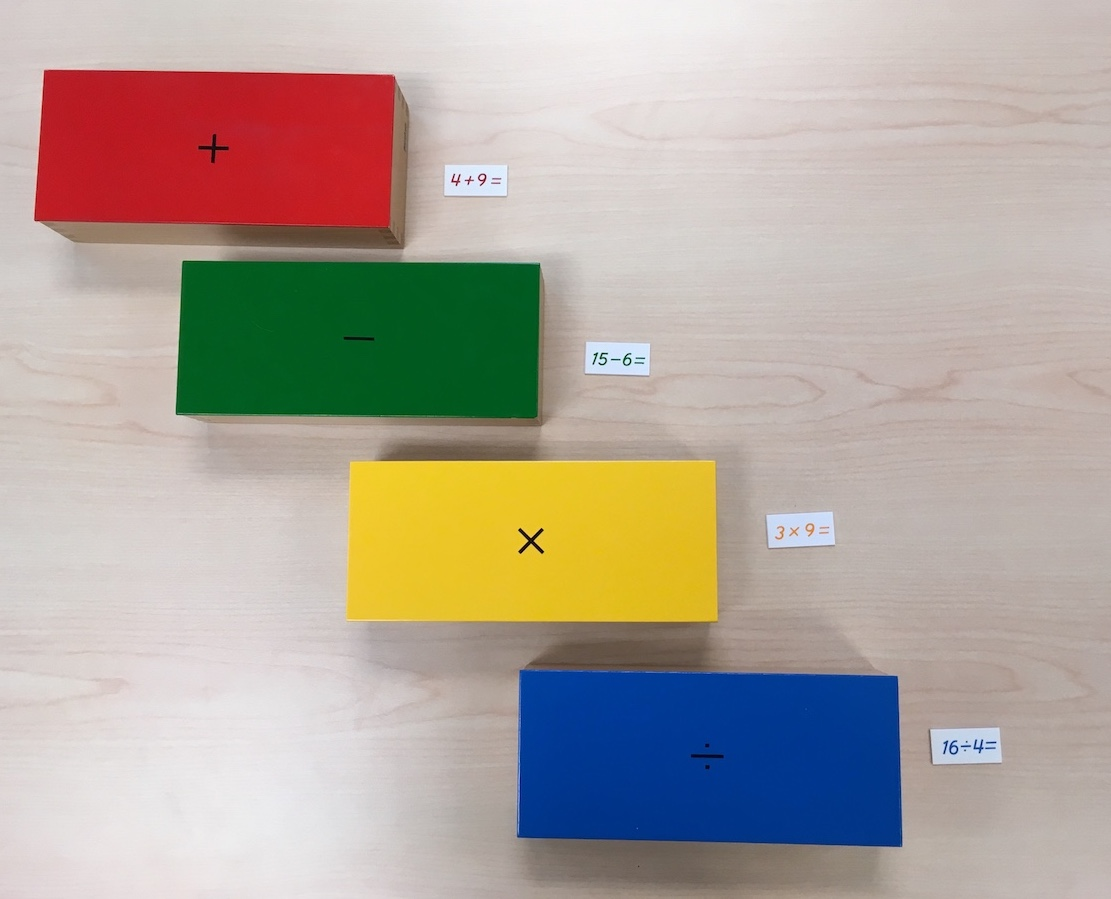# Memorization

Explore activities that help children memorize addition, subtraction, multiplication, and division math facts.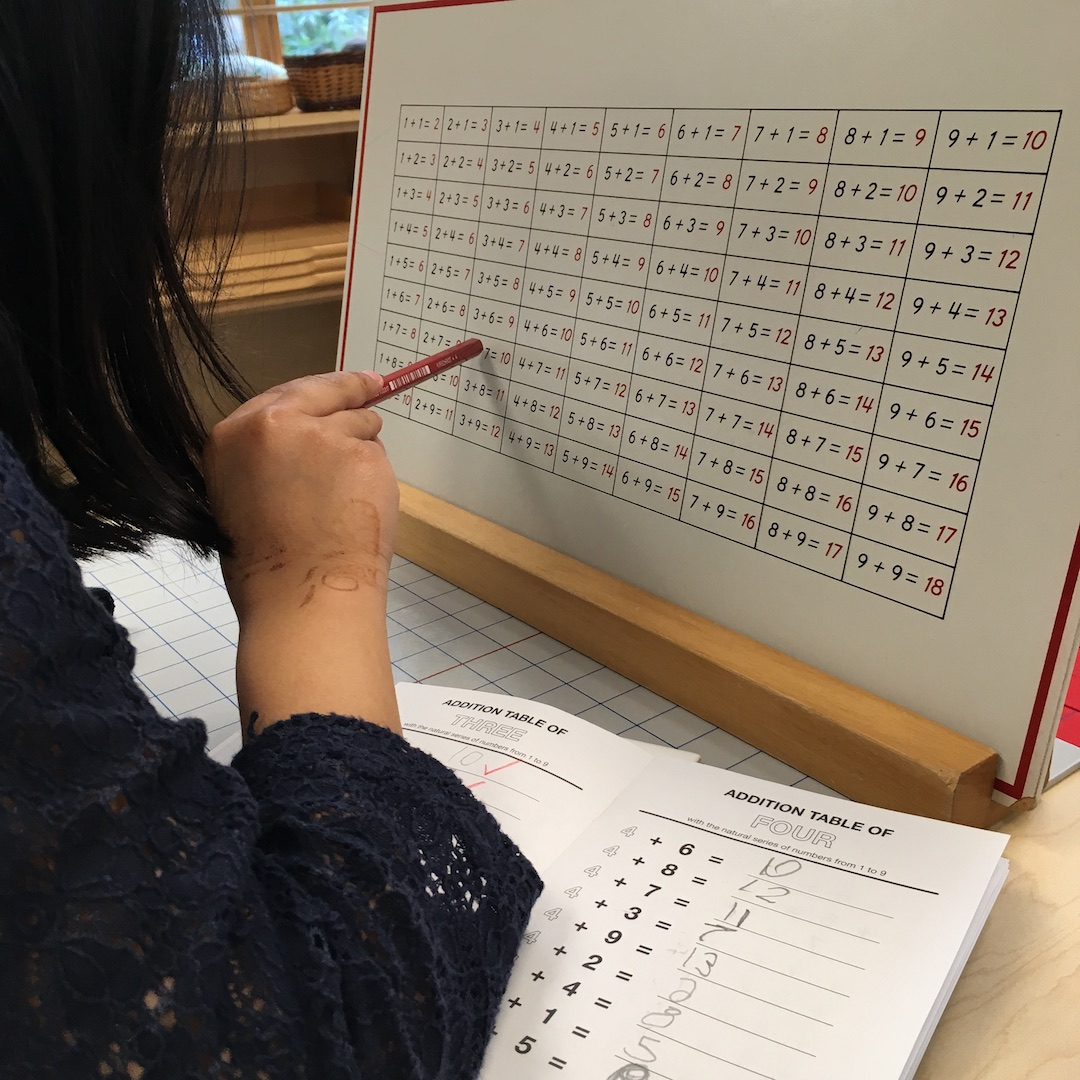Memorization is:

“The process of committing something to memory or learning something by heart.”

-Oxford dictionary.

Memorizing math facts is something that all students work towards during their time in primary and elementary school. Children engage in repetitive exercises to learn 5 + 5 =10 and 8 – 5 = 3. Primary Montessori children work on memorizing these same essential equations. However, in the Montessori approach, we first introduce children to the big-picture concept of the four operations so that they have an understanding of what 5+5 actually means. Children use hands-on materials to learn that addition is “putting together small quantities to make a larger quantity.” They play games to determine that multiplication is “putting the same quantity together many times to yield a larger quantity.” Only after lots of experience with these big-picture concepts do we introduce the memorization of essential math facts. The children then find that learning these equations by heart helps them to perform the four operations with greater accuracy.

## At Home Memorization Activities:

In the Montessori classroom, we introduce children to hand-on materials that help them derive the math facts. The activities below are opportunities for repetition with memorization after these hands-on lessons have been practiced.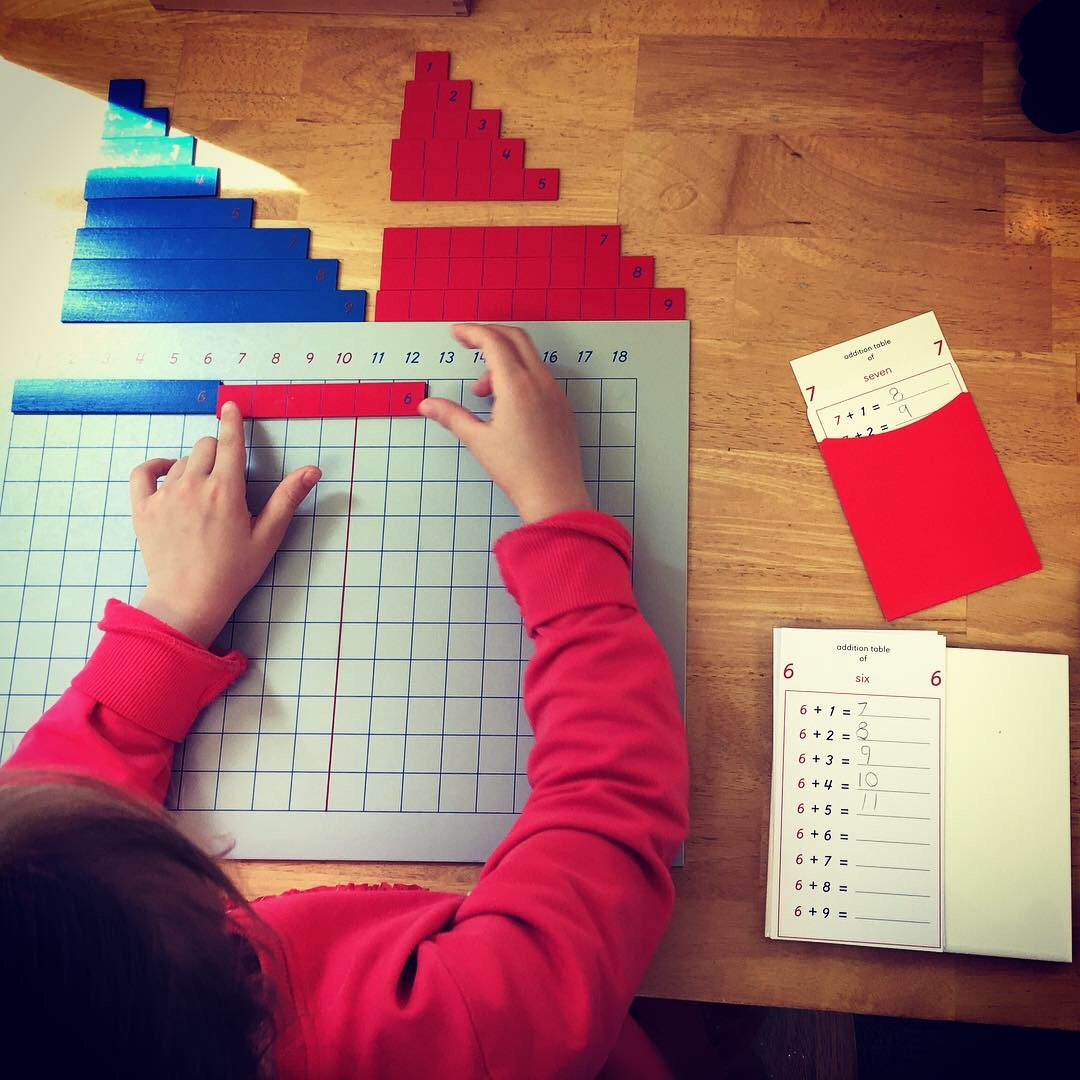### Addition Strip Board ages 5 -5 ½ and up

Directions: Print the addition strip board and strips on cardstock. Cut out the red and blue addition strips. Print the addition tables. To get started, place the blue strips in order above the left side of the chart. Place the red strips in order above the right side of the chart. Read the first equation listed on the addition table. Use one blue strip and one red strip to find the answer to the equation. Record the answer on the table. Continue until you have explored all tables 1-9. Use the control chart to check your answers. When you are finished, staple your equation sheets together to make an addition booklet. Over time, progress to the second set of addition tables in which the equations are mixed.

* The addition stripboard is best used after a formal presentation by the teacher.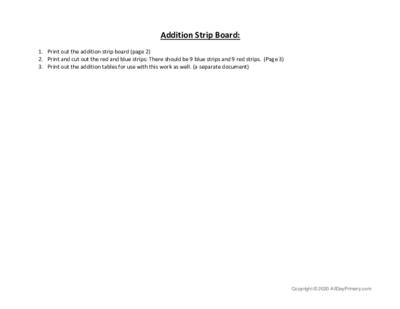addition strip board.pdf 106 KB Download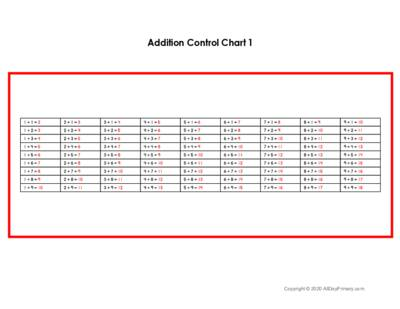Addition Control Chart 1.pdf 101 KB Download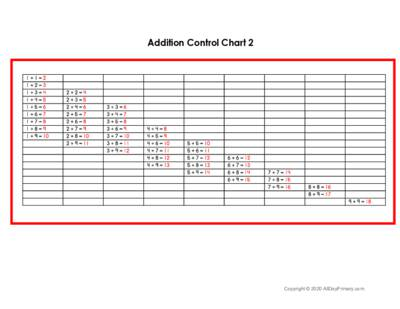Addition Control Chart 2.pdf 244 KB Download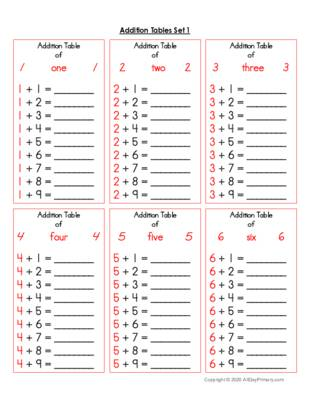Addition Tables Set 1.pdf 196 KB Download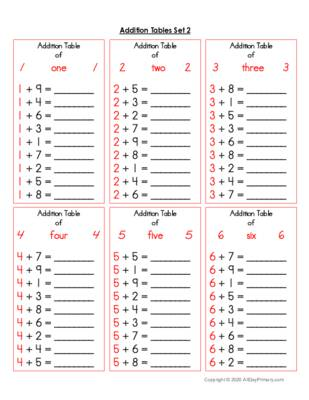Addition Tables Set 2.pdf 197 KB Download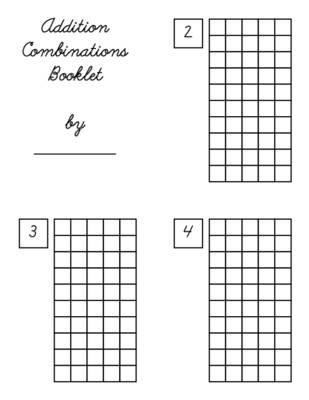Addition Combinations Booklets for Home.pdf 56 KB Download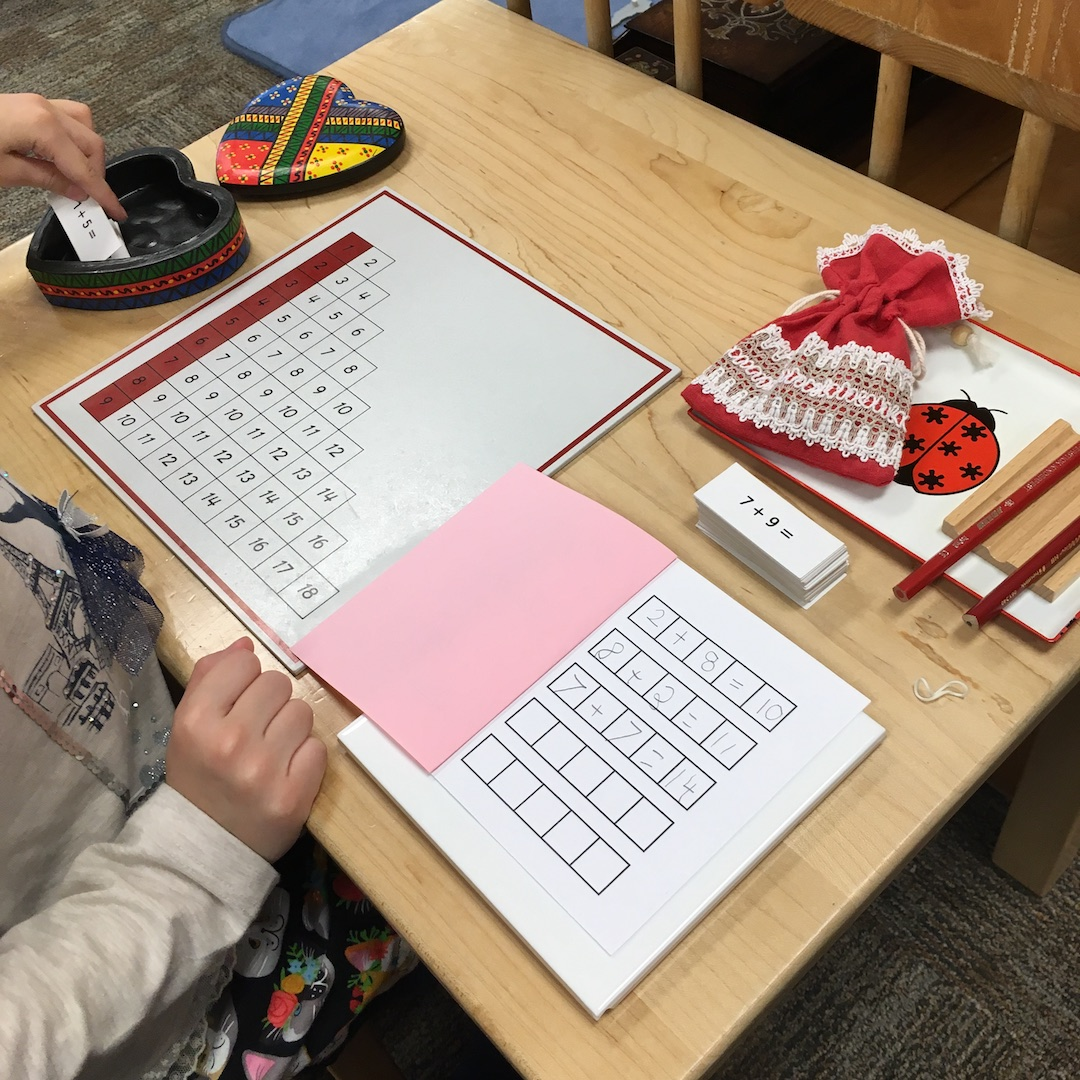### Addition Practice Charts ages 5 -5 ½ and up

Directions: Print the addition practice chart. Print and cut out the addition equations. Read each essential addition equation. Try to determine the answer without using your fingers or counting in your head. If the answer pops into your mind immediately, you know this equation by heart. Otherwise, use the practice chart to solve the addition equation. Record any unknown equations on the math paper below and repeat the full equation with the answer (8 + 8 =16) to help with retention. Continue until you have explored all 72 equations. Use the control chart to check your answers to ensure you are memorizing the correct equation. When you are finished, staple your equation sheets together to make an addition booklet. Over time, you will not have to use the chart at all!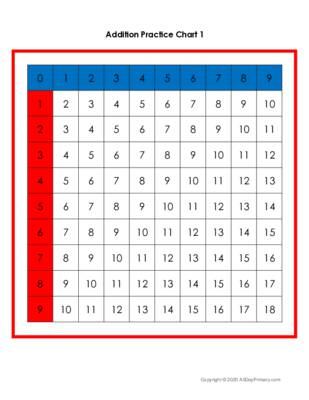Addition Chart 1.pdf 40 KB Download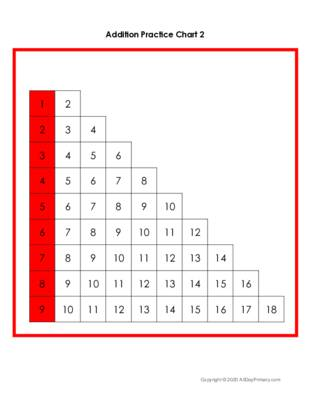Addition Chart 2.pdf 38 KB Download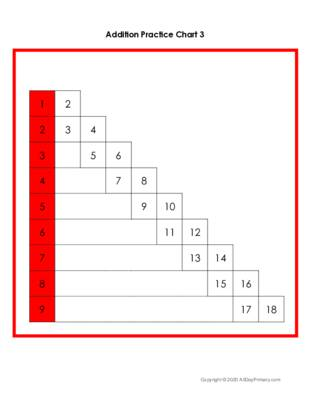Addition Chart 3.pdf 43 KB Download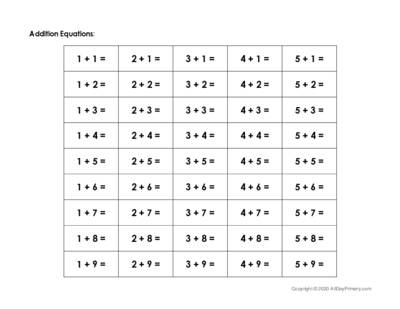Addition Equations.pdf 38 KB DownloadAddition Control Chart 1.pdf 101 KB DownloadAddition Control Chart 2.pdf 244 KB Download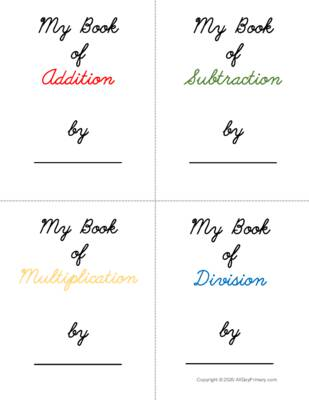Math Booklet Covers.pdf 25 KB Download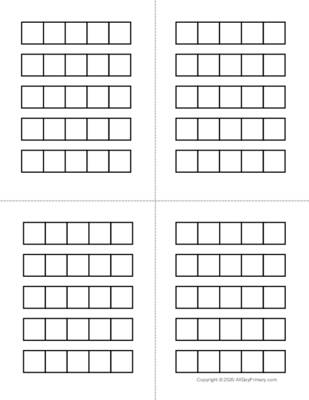Math Equation Booklet Inserts.pdf 20 KB Download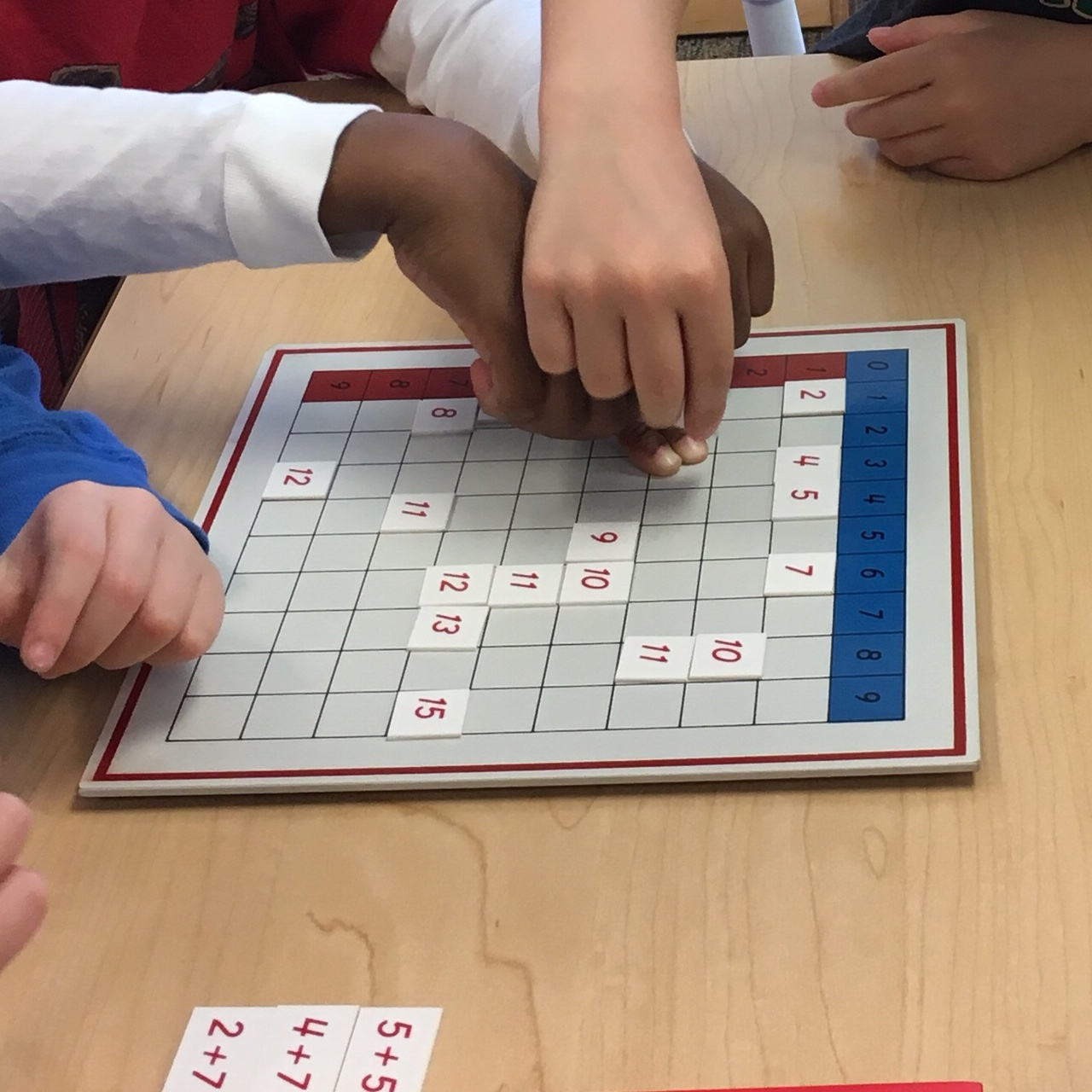### Addition Blank Chart ages 5 -5 ½ and up

Directions: Print the addition blank chart and the addition answer tiles. Cut out the tiles and arrange them above the blank chart, grouping like numbers. Print and cut out the addition equations. Read each essential addition equation and determine the answer. Look for the answer tile. Use your fingers to find where the answer tile should be placed on the blank chart (similar to how you used the addition practice chart 1). Continue until the entire chart has been completed, and all of the answer tiles have been placed.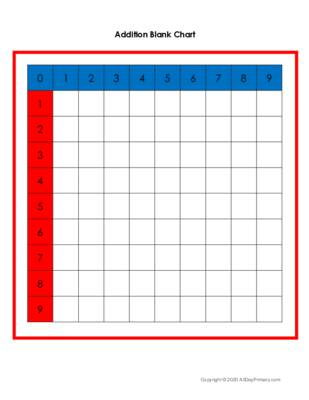Addition Blank Chart.pdf 49 KB DownloadAddition Equations.pdf 38 KB Download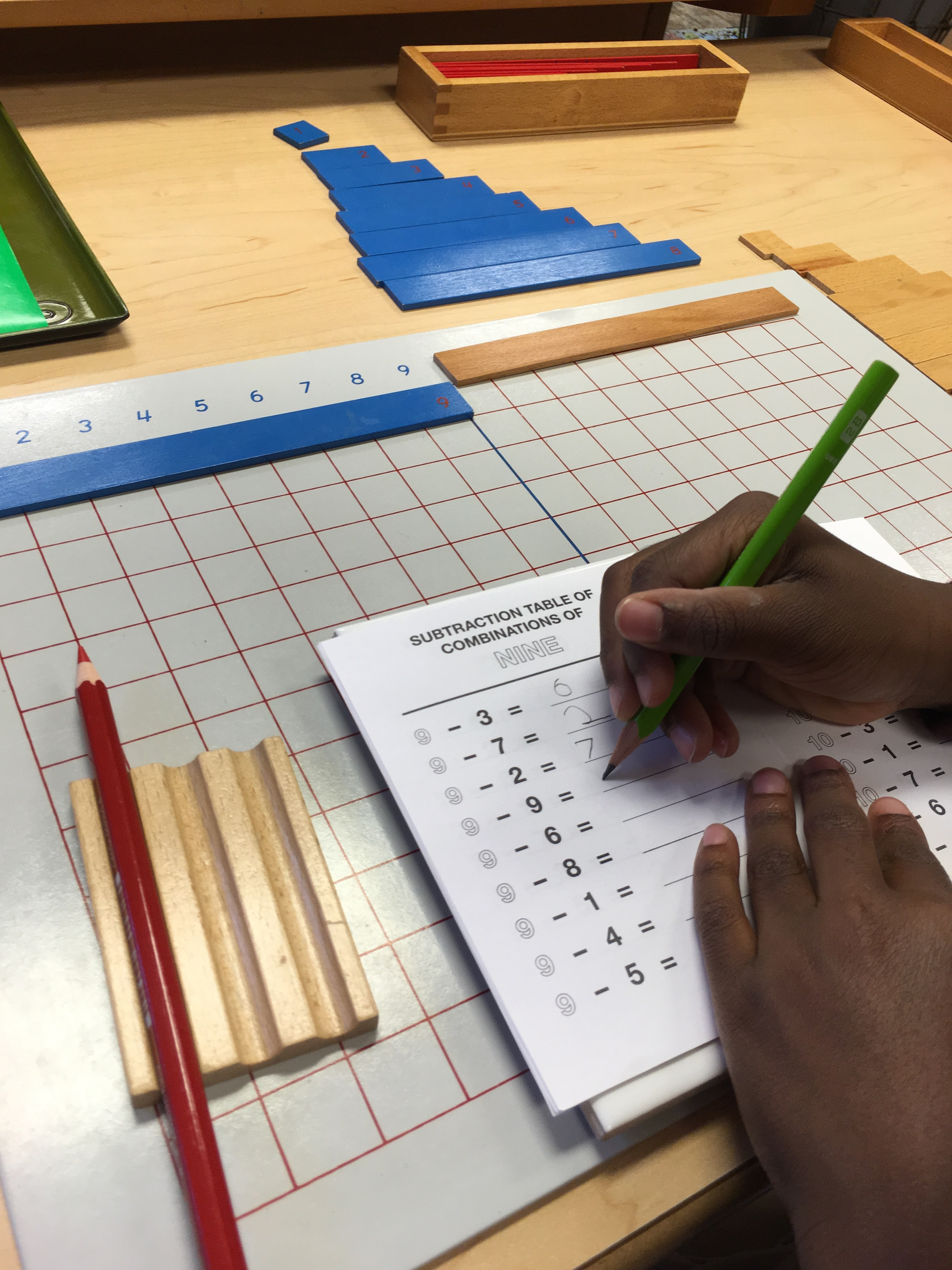### Subtraction Strip Board ages 5 -5 ½ and up

Notes: The Subtraction Strip Board is a bit more involved than the addition strip board and is best used after a formal presentation by the teacher. Please see your child’s guide for full instructions as to how to use this material.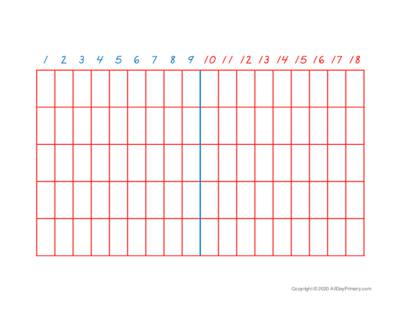subtraction strip board.pdf 424 KB Download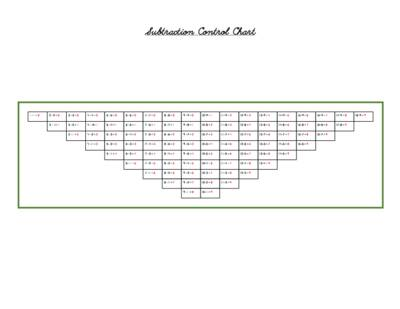Subtraction Control Chart.pdf 94 KB Download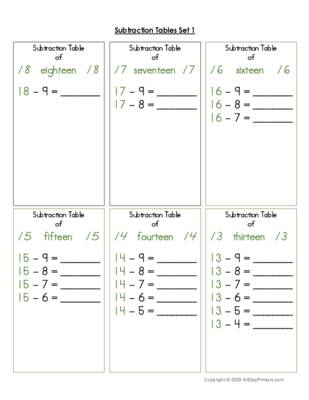Subtraction Tables Set 1.pdf 361 KB Download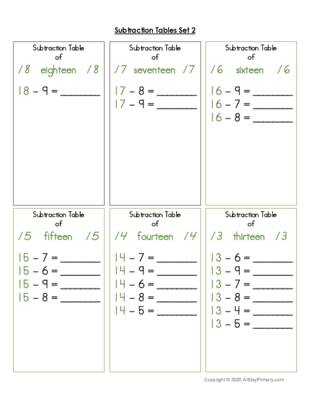Subtraction Tables Set 2.pdf 361 KB Download

### Subtraction Practice Chart ages 5 ½ and up

Directions: Print the subtraction practice chart. Print and cut out the subtraction equations. Read each essential subtraction equation. Try to determine the answer without using your fingers or counting in your head. If the answer pops into your mind immediately, you know this equation by heart. Otherwise, use the practice chart to solve the subtraction equation. Record the equation on the math paper below and repeat the full equation with the answer (18 - 9= 9) to help with retention. Continue until you have explored all equations. Use the control chart to check your answers to ensure you are memorizing the correct equation. When you are finished, staple your equation sheets together with a cover sheet to make a subtraction booklet. Over time, you will not have to use the chart at all!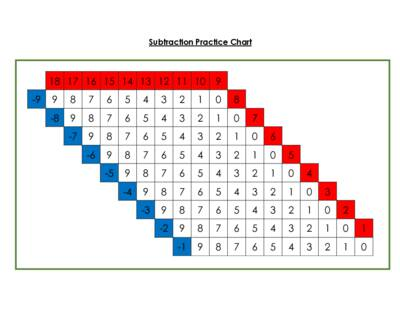Subtraction Practice Chart.pdf 31 KB Download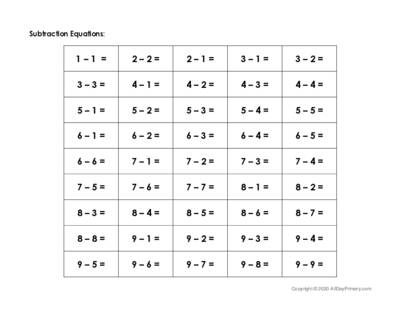Subtraction Equations.pdf 40 KB DownloadSubtraction Control Chart.pdf 94 KB DownloadMath Booklet Covers.pdf 25 KB DownloadMath Equation Booklet Inserts.pdf 20 KB Download

### Subtraction Blank Chart ages 5 ½ and up

Directions: Print the subtraction blank chart and the subtraction answer tiles. Cut out the tiles and arrange them above the blank chart, grouping like numbers. Print and cut out the subtraction equations. Read each essential subtraction equation and determine the answer. Look for the answer tile. Use your fingers to find where the answer tile should be placed on the blank chart (similar to how you used the subtraction practice chart). Continue until the entire chart has been completed, and all of the answer tiles have been placed.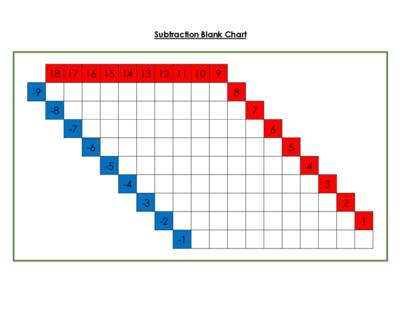Subtraction Blank Chart.pdf 40 KB DownloadSubtraction Equations.pdf 40 KB Download

### Number Puzzles ages 6 and up

Directions: Use your addition and subtraction math facts to solve the number puzzle.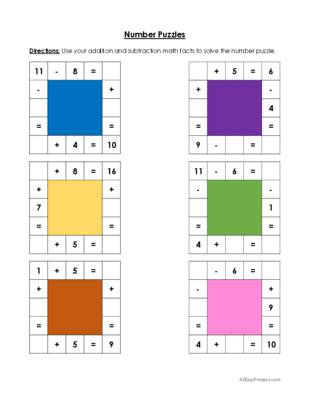Number Puzzles.pdf 44 KB Download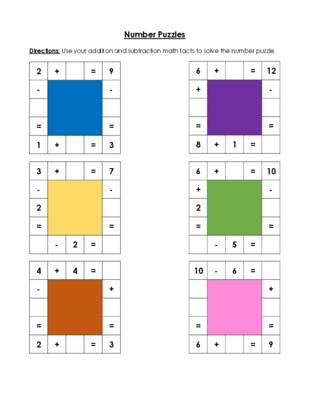Number Puzzles 2.pdf 35 KB Download

###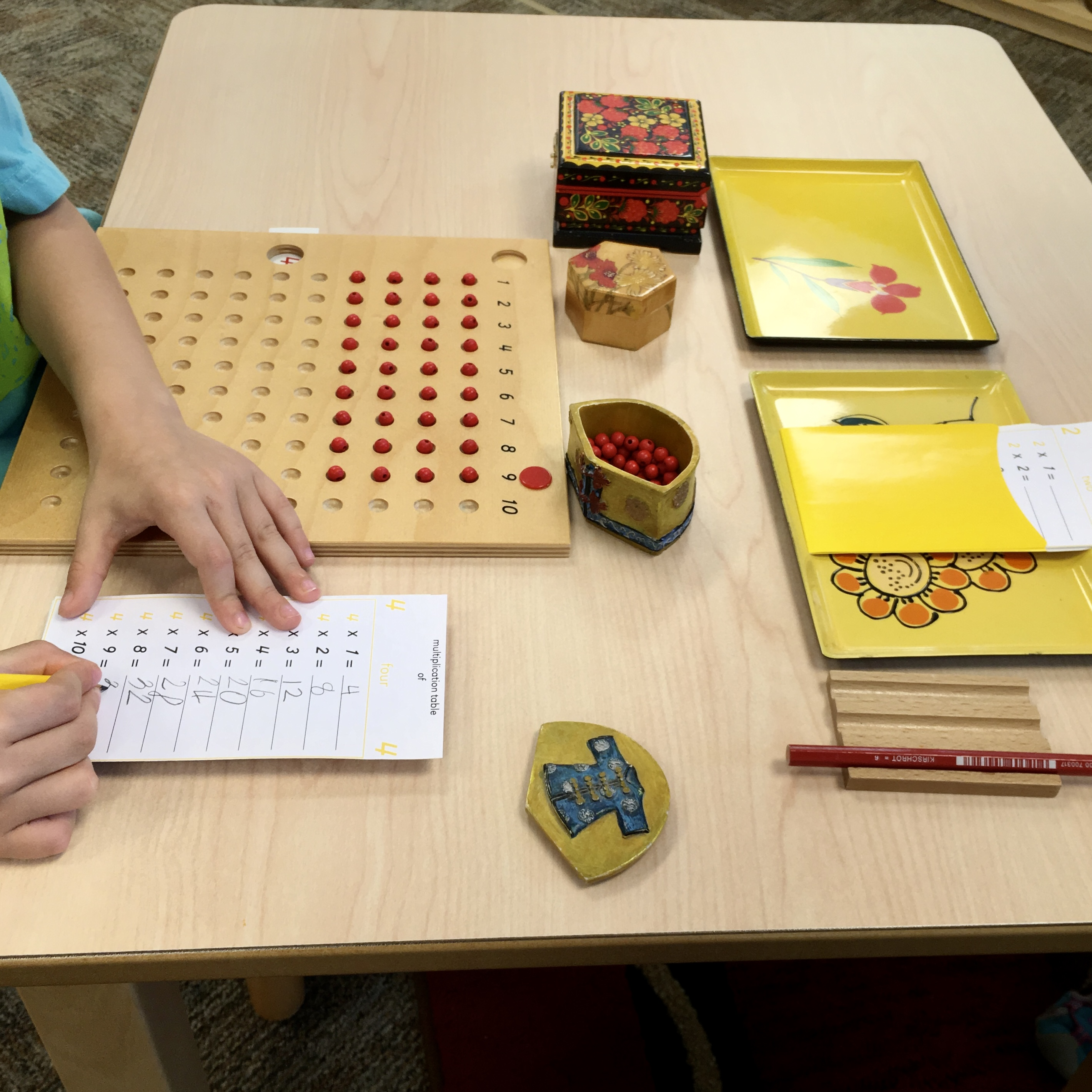Notes: The Multiplication Board is a bit more involved than many of the other memorization materials and is best used after a formal presentation by the teacher. Please see your child’s guide for full instructions as to how to use this material.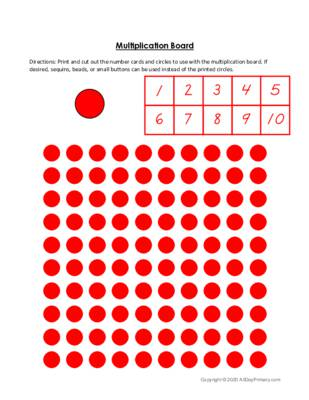Multiplication Board.pdf 86 KB Download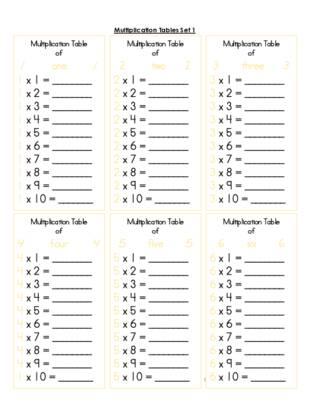Multiplication Tables Set 1.pdf 208 KB Download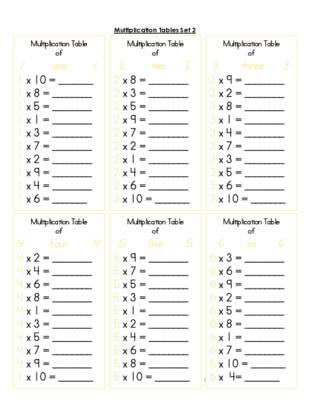Multiplication Tables Set 2.pdf 210 KB Download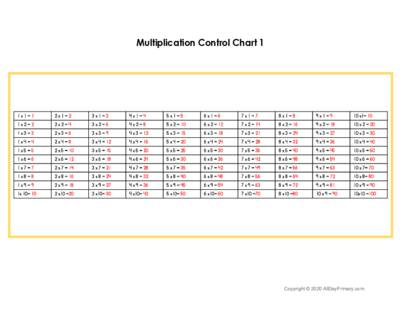Multiplication Control Chart-1.pdf 120 KB Download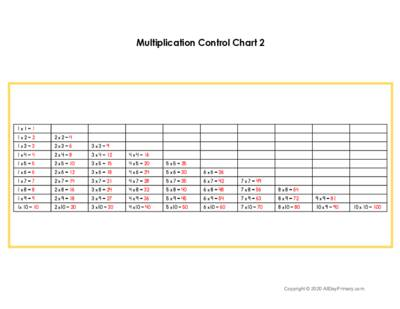Multiplication Control Chart-2.pdf 117 KB Download

### Multiplication By Ten ages 5 ½ and up

Notes:  After working with the multiplication bead bars, children learn the pattern that occurs when a number is taken ten times. This allows them to quickly solve problems involving any number multiplied by 10.

Directions: Print the worksheet and solve the multiplication problems. Use commas as needed as you record the answer. Read the answer aloud as a composite number (13,254 = Thirteen Thousand, two hundred fifty-four).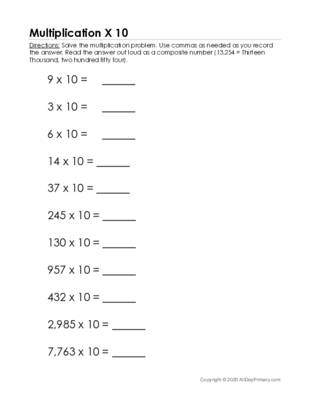Multiplication X 10.pdf 41 KB Download

### Multiplication Practice Charts ages 5 ½ and up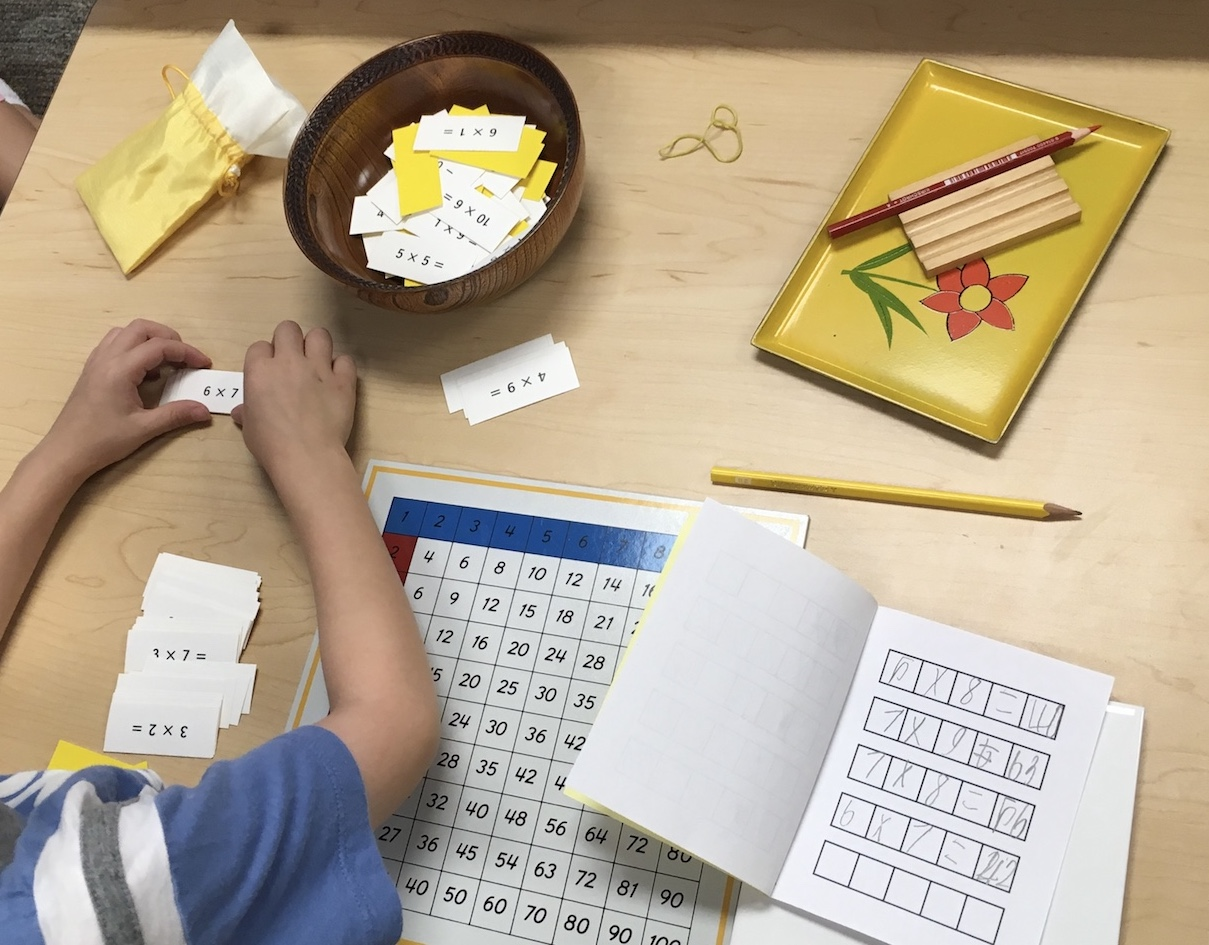Directions: Print the multiplication practice chart. Print and cut out the multiplication equations. Read each essential multiplication equation. Try to determine the answer without using your fingers or counting in your head. If the answer pops into your mind immediately, you know this equation by heart. Otherwise, use the practice chart to solve the multiplication equation. Record any unknown equations on the math paper below and repeat the full equation with the answer (8 x 8 =64) to help with retention. Continue until you have explored all of the equations. Use the control chart to check your answers to ensure you are memorizing the correct equation. When you are finished, staple your equation sheets together to make a multiplication booklet. Over time, you will not have to use the chart at all!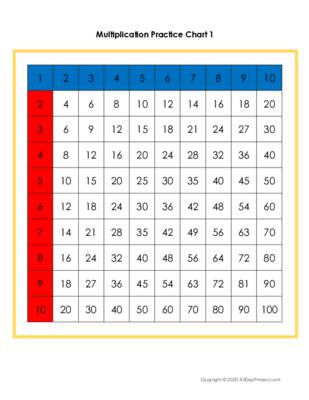Multiplication Practice Chart 1.pdf 40 KB DownloadMultiplication Practice Chart 2.pdf 39 KB DownloadMultiplication Control Chart-2.pdf 117 KB Download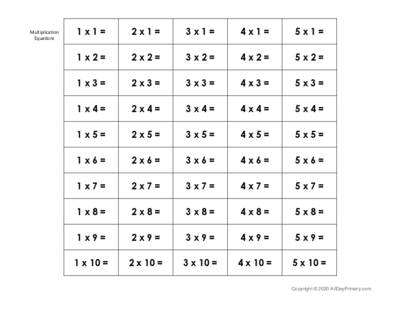Multiplication Equations.pdf 42 KB DownloadMath Booklet Covers.pdf 25 KB DownloadMath Equation Booklet Inserts.pdf 20 KB Download

### Multiplication Blank Chart ages 6 and up

Directions: Print the multiplication blank chart and the multiplication answer tiles. Cut out the tiles and arrange them above the blank chart, grouping like numbers. Print and cut out the multiplication equations. Read each essential equation and determine the answer. Look for the answer tile. Use your fingers to find where the answer tile should be placed on the blank chart (similar to how you used the multiplication practice chart 1). Continue until the entire chart has been completed, and all of the answer tiles have been placed.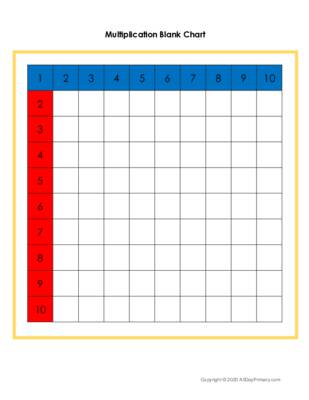Multiplication Blank Chart.pdf 47 KB DownloadMultiplication Equations.pdf 42 KB Download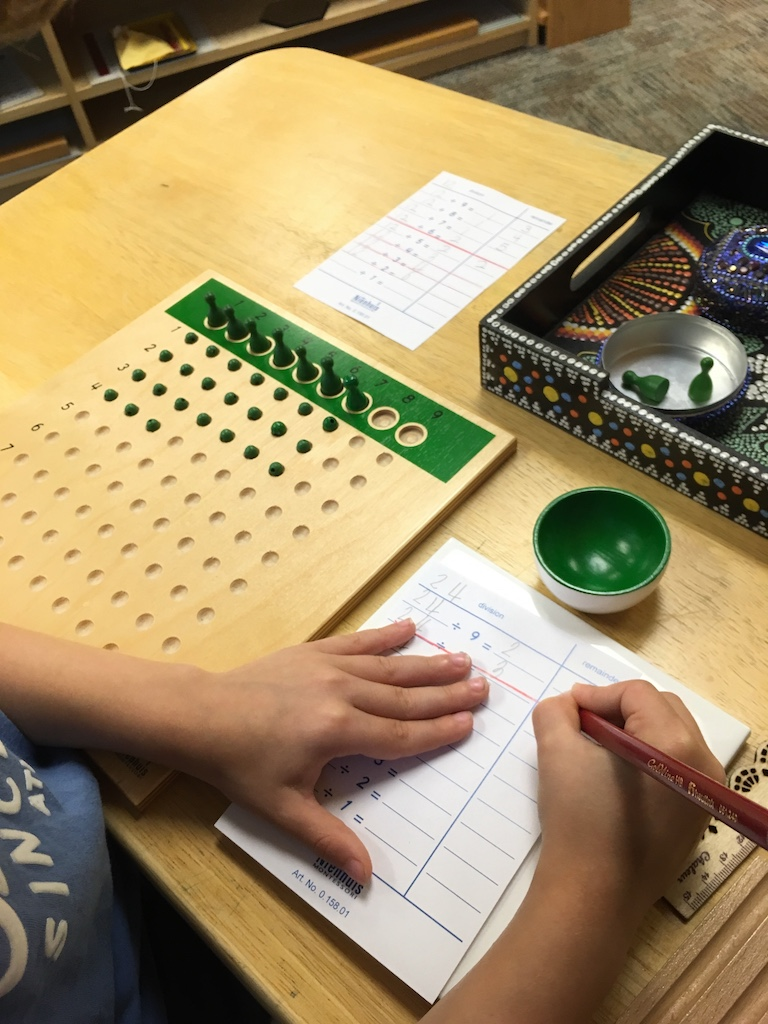### The Unit Division Board ages 6 and up

Notes: The Unit Division Board is used to help children derive the essential division equations. This lesson is a bit more involved than many of the other memorization materials and is best used after a formal presentation by the teacher. Please see your child’s guide for full instructions as to how to use this material.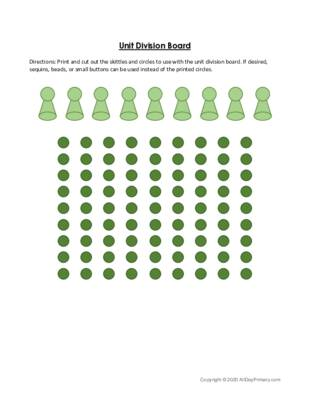Unit Division Board.pdf 75 KB Download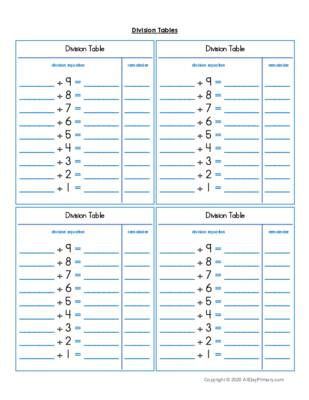Division Tables.pdf 145 KB Download

### Division Practice Chart ages 6 and up

Directions: Print the division practice chart. Print and cut out the division equations. Read each essential division equation. Try to determine the answer without using your fingers or counting in your head. If the answer pops into your mind immediately, you know this equation by heart. Otherwise, use the practice chart to solve the division equation. Record any unknown equations on the math paper below and repeat the full equation with the answer (64 / 8 =8) to help with retention. Continue until you have explored all of the equations. Use the control chart to check your answers to ensure you are memorizing the correct equation. When you are finished, staple your equation sheets together to make a multiplication booklet. Over time, you will not have to use the chart at all!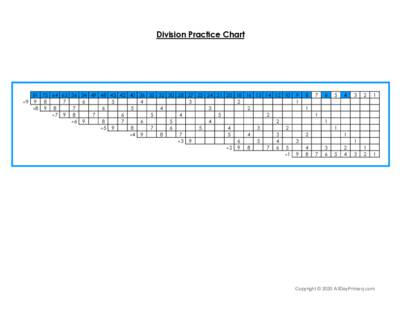Division Practice Chart.pdf 43 KB Download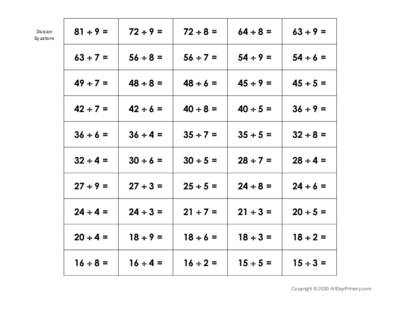Division Equations.pdf 44 KB Download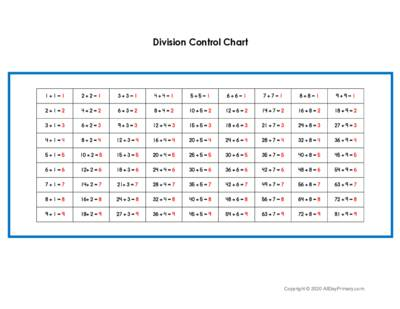Division Control Chart.pdf 44 KB DownloadMath Booklet Covers.pdf 25 KB DownloadMath Equation Booklet Inserts.pdf 20 KB Download